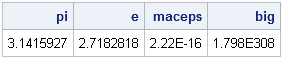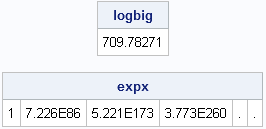Statistical programmers often need mathematical constants such as π (3.14159...) and e (2.71828...). Programmers of numerical algorithms often need to know machine-specific constants such as the machine precision constant (2.22E-16 on my Windows PC) or the largest representable double-precision value (1.798E308 on my Windows PC).

Some computer languages build these constants into the language as reserved (or semi-reserved) words. This can lead to problems. For example, statisticians use the symbol pi to represent mixture probabilities in mixture distributions. In some languages, you can assign the symbol pi to a vector, which overrides the built-in value and causes cos(pi) to have an unexpected value. In other languages, the symbol pi is a reserved keyword and is unavailable for assignment.

In SAS, constants are not built into the language. Instead, they are available through a call to the CONSTANT function. You can call the CONSTANT function from the DATA step, from SAS/IML programs, and from SAS procedures (such as PROC FCMP, PROC MCMC, and PROC NLIN) that enable you to write SAS statements inside the procedure.

For example, the following SAS/IML statements get two mathematical and two machine-specific constant and assign them to program variables:

```proc iml; pi = constant("pi"); e = constant("e"); maceps = constant("maceps"); big = constant("big"); print pi e maceps big;```The constants are printed by using the default BEST9. format; you can use a format such as BEST16. to see that the values contain additional precision. For example, the value that is actually stored in the pi variable is 3.14159265358979.

Most programmers understand the need for constants like "pi." However, I often use machine-specific constants in order to write robust numerical algorithms that avoid numerical overflow and underflow. For example, suppose that you have some data and you want to apply an exponential transformation to the data. (In other word, you want to compute exp(x) for each value of x.) The exponential function increases very quickly, so exp(x) cannot be stored in a double-precision value when x is moderately large. As a result, the following computation causes a numerical overflow error:

```x = do(0, 1000, 200); /* {0, 200, 400, ..., 1000} */ expx = exp(x); /* ERROR: numerical overflow */```
```ERROR: (execution) Invalid argument to function.

count     : number of occurrences is 2
operation : EXP at line 2154 column 11
operands  : x

<...more error information (omitted)..>
```

The largest value of x that can be exponentiated is found by calling the CONSTANT function with the "LOGBIG" argument. You can use the "LOGBIG" value to locate values of x that are too large to be transformed. You can then assign missing values to exp(x) for those "large" values of x, as shown in the following statements:

```logbig = constant("logbig"); idx = loc(x<logbig); /* locate values for which exp(x) does not overflow */ expx = j(1,ncol(x), .); /* allocate result vector of missing values */ expx[idx] = exp( x[idx] ); /* exp(x) only for "sufficiently small" x */ print logbig, expx;```In this way, you can write robust code that does not fail when there are large values in the data. Similarly, you can use this idea to protect against overflow when raising a value to a power by using the '**' operator in the DATA step or the '##' operator in SAS/IML.

This blog post was inspired by a tweet from @RLangTip on Twitter: "Constants built into #rstats: letters, months and pi," which linked to documentation on constants that are built into the R language.

ShareDistinguished Researcher in Computational Statistics

Rick Wicklin, PhD, is a distinguished researcher in computational statistics at SAS and is a principal developer of SAS/IML software. His areas of expertise include computational statistics, simulation, statistical graphics, and modern methods in statistical data analysis. Rick is author of the books Statistical Programming with SAS/IML Software and Simulating Data with SAS.

1.2.